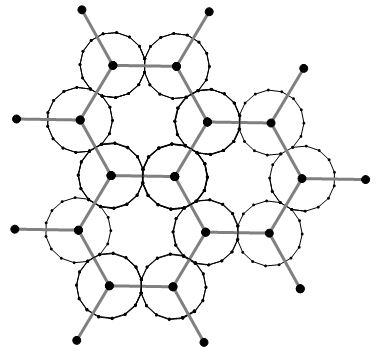## Monday, May 16, 2016

### some tilings on regular grids

The only regular polygons that can tile the plane by themselves are equilateral triangles, squares, and regular hexagons (these are the regular tilings).

In the previous post, we noticed that the regular hexagon tiling can be used as a basis for creating decorative tilings of other polygons, such as nonagons, decagons, 15-gons, and so on, that include increasingly curved (but still polygonal) six-pointed stars to fill in the gaps, observing with the help of a formula form another post, that polygons that can be arranged around a regular hexagon like this have a number of sides equal to a multiple of 3.15-gons on a regular hexagonal grid

We can do the same sort of thing with the regular triangle tiling, arranging hexagons, dodecagons, 18-gons, or any n-gon where n is a multiple of 6, and the gaps become increasingly-circular triangles.

And the same with the square tiling, arranging squares, octagons, dodecagons, 16-gons, or any n-gon where n is a multiple of 4, where the gaps becoming somewhat astroidal.

The polygons that can be arranged on the triangular, square and hexagonal grids can all be found by looking at the intersection of the green horizontal lines with the family of curves below, which as explained in an earlier post, describe a "regular polygons-in-rings formula" k = 2n/(n -(2+2m)).

The  regular polygon with the fewest number of sides that can be arranged this way on all of these grids is the dodecagon (some other decorative tilings from dodecagons here)

The image below shows where some of these tilings sit on the k = 2n/(n -(2+2m)) curve family.

## Friday, May 13, 2016

### a tiling of regular nonagons and stars

When I found this nice nonagon and star tiling, I couldn't get They Might Be Giants out of my head. The underlying symmetry comes from hexagons though:

The relationship described in the previous post tells us that the next regular polygon that can be put around a hexagon like this is the dodecagon (see the line k = 6):

And sure enough, these dodecagons can also form a nice hexagon-based tiling, with somewhat pointier stars.

The k = 6 line above gives us a whole family of polygon and star hexagonal tilings that follow the relationship n = 3m + 3 - the stars don't start to appear until m = 2